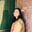Trusted answers to developer questions
Trusted Answers to Developer Questions

Related Tags

numpy
python

# What is numpy.log() in Python?Umme Ammara

Grokking Modern System Design Interview for Engineers & Managers

Ace your System Design Interview and take your career to the next level. Learn to handle the design of applications like Netflix, Quora, Facebook, Uber, and many more in a 45-min interview. Learn the RESHADED framework for architecting web-scale applications by determining requirements, constraints, and assumptions before diving into a step-by-step design process.

Python’s numpy.log() is a mathematical function that computes the natural logarithm of an input array’s elements.

The natural logarithm is the inverse of the exponential function, such that log (exp(x)) = x.

## Syntax

numpy.log is declared as shown below:

numpy.log(x, /, out=None, *, where=True, casting='same_kind', order='K', dtype=None, subok=True[, signature, extobj]) = <ufunc 'log'>


In the syntax above, x is the non-optional parameter and the rest are optional parameters.

A universal function (ufunc) is a function that operates on ndarrays in an element-by-element fashion. The log() method is a universal function.

## Parameters

The numpy.log() method takes the following compulsory parameter:

• x [array-like] - input array.

The numpy.log() method takes the following optional parameters:

 Parameter Description out represents the location into which the output of the method is stored. where True value indicates that a universal function should be calculated at this position. casting controls the type of datacasting that should occur. The same_kind option indicates that safe casting or casting within the same kind should take place. order controls the memory layout order of the output function. The option K means reading the elements in the order they occur in memory. dtype represents the desired data type of the array. subok decides if subclasses should be made or not. If True, subclasses will be passed through.

## Return value

numpy.log() returns the element-wise natural logarithm of values in x. If x is scalar, the return type is also scalar.

• If x is a real-valued data type, the return type will also be a real value. If a value cannot be written as a real value, then NaN is returned.

• If x is a complex-valued input, the numpy.log method has a branch cut [-inf,0], and it is continuous above it.

## Examples

In the example below, numpy.log() computes the natural logarithm of two numbers, a and b:

import numpy as npa = 0.5 b = np.exp(8.9)print (np.log(a))print (np.log(b))

In the example below, numpy.log() computes the element-wise natural logarithm of the array arr1:

import numpy as nparr1 = np.array([1,2,0,3,4])print (np.log(arr1))

RELATED TAGS

numpy
python

CONTRIBUTORUmme Ammara